×#### Thank you for registering.

One of our academic counsellors will contact you within 1 working day.

Click to Chat

1800-1023-196

+91-120-4616500

CART 0

• 0

MY CART (5)

Use Coupon: CART20 and get 20% off on all online Study Material

ITEM
DETAILS
MRP
DISCOUNT
FINAL PRICE
Total Price: Rs.

There are no items in this cart.
Continue Shopping• Complete JEE Main/Advanced Course and Test Series
• OFFERED PRICE: Rs. 15,900
• View Details

Chapter 9: Linear Equation in One Variable Exercise – 9.2

Question: 1

Solve each of the following equations and also verify your solutions: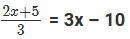Solution:Question: 2

Solve each of the following equations and also verify your solutions: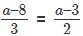Solution: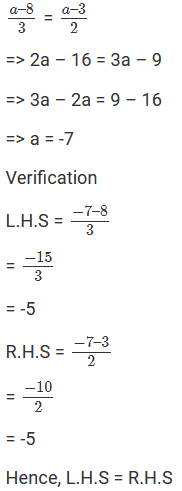Question: 3

Solve each of the following equations and also verify your solutions:Solution: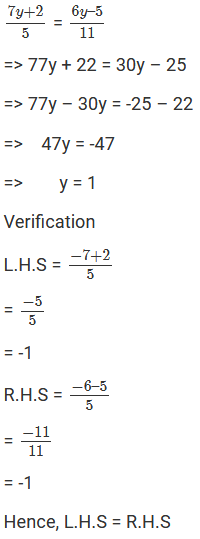Question: 4

Solve each of the following equations and also verify your solutions: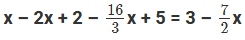Solution:Question: 5

Solve each of the following equations and also verify your solutions:Solution: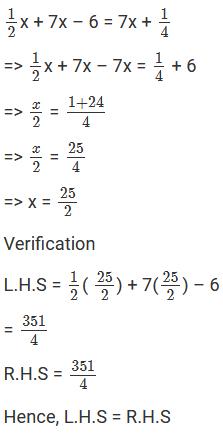Question: 6

Solve each of the following equations and also verify your solutions: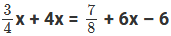Solution: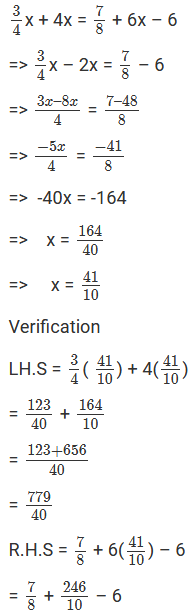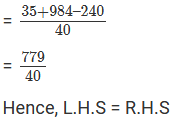Question: 7

Solve each of the following equations and also verify your solutions: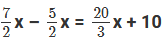Solution:Question: 8

Solve each of the following equations and also verify your solutions: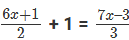Solution: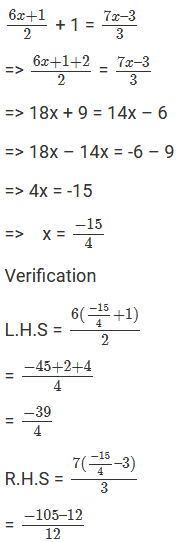= −39/4

Hence, L.H.S = R.H.S

Question: 9

Solve each of the following equations and also verify your solutions: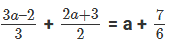Solution: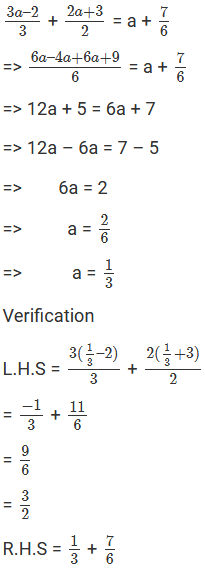= 9/6

= 3/2

Question: 10

Solve each of the following equations and also verify your solutions:Solution: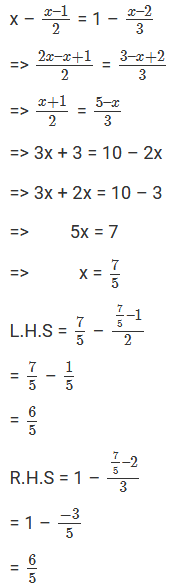Hence, L.H.S = R.H.S

Question: 11

Solve each of the following equations and also verify your solutions: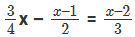Solution: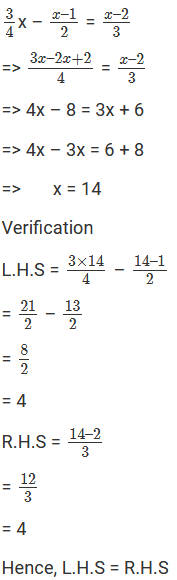Question: 12

Solve each of the following equations and also verify your solutions:Solution: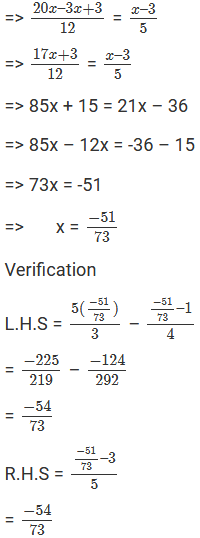Hence, L.H.S = R.H.S

Question: 13

Solve each of the following equations and also verify your solutions: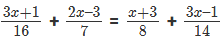Solution: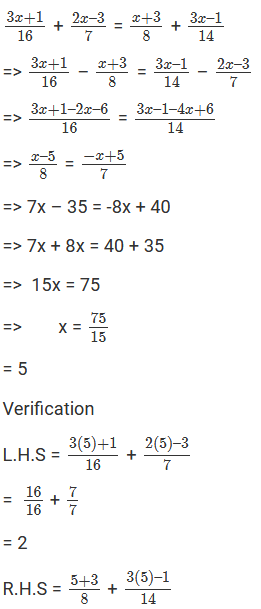Question: 14

Solve each of the following equations and also verify your solutions: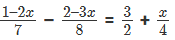Solution: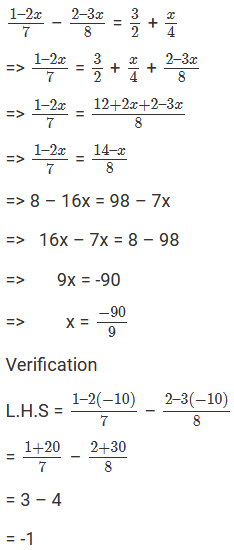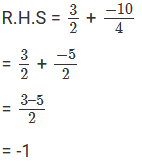Question: 15

Solve each of the following equations and also verify your solutions: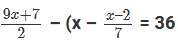Solution: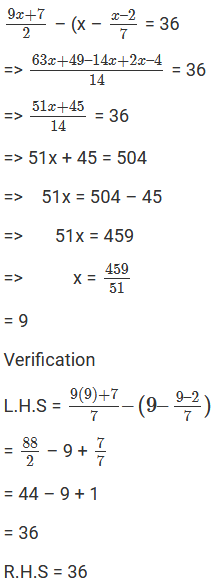Hence, L.H.S = R.H.S

Question: 16

Solve each of the following equations and also verify your solutions:

0.18(5x – 4) = 0.5x + 0.8

Solution:

0.18(5x – 4) = 0.5x + 0.8

=> 0.9x – 0.72 = 0.5x + 08

=> 0.9x – 0.5x = 0.8 + 0.72

=> 0.4x = 1.52

=> x = 1.52/0.4

= 3.8

Verification

L.H.S = 0.18(5(3.8) – 4)

= 0.18 × 15

= 2.7

R.H.S = 0.5(3.8) + 0.8

= 2.7

Hence, L.H.S = R.H.S

Question: 17

Solve each of the following equations and also verify your solutions: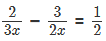Solution: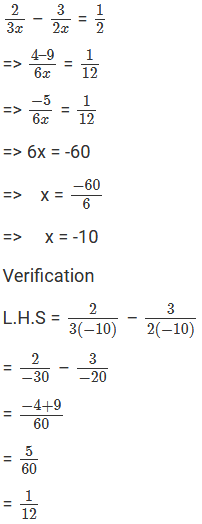R.H.S = 1/12

Hence, L.H.S = R.H.S

Question: 18

Solve each of the following equations and also verify your solutions: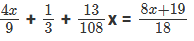Solution: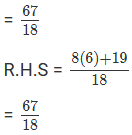Question: 19

Solve each of the following equations and also verify your solutions:Solution: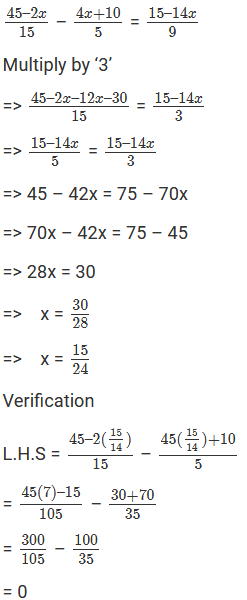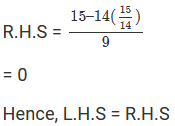Question: 20

Solve each of the following equations and also verify your solutions: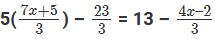Solution: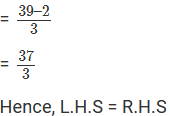Question: 21

Solve each of the following equations and also verify your solutions:Solution:Question: 22

Solve each of the following equations and also verify your solutions: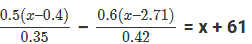Solution: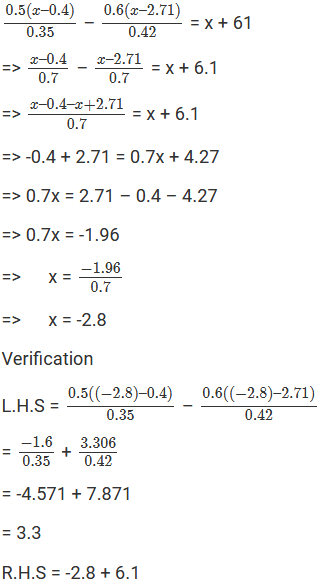= 3.3

Hence, L.H.S = R.H.S

Question: 23

Solve each of the following equations and also verify your solutions: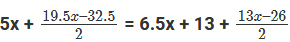Solution: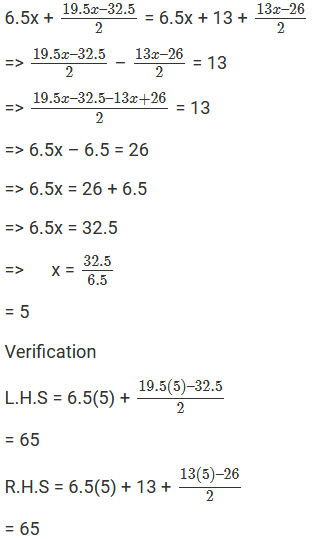Hence, L.H.S = R.H.S

Question: 24

Solve each of the following equations and also verify your solutions:

(3x – 8) (3x + 2) – (4x – 11) (2x + 1) = (x – 3) (x + 7)

Solution:

(3x – 8) (3x + 2) – (4x – 11) (2x + 1) = (x – 3) (x + 7)

=> 9x2 + 6x – 24x – 16 – 8x2 – 4x + 22x + 11 = x2 + 7x – 3x – 21

=> x2 – 5 = x2 + 4x – 21

=> 4x = 21 – 5

=>  4x = 16

=> x = 164

= 4

Verification

L.H.S = (3(4) – 8) (3(4) + 2) – (4(4) – 11) (2(4) + 1)

= 4(16) – 5(9)

= 11

R.H.S = (4 – 3) (4 + 7)

= 11

Hence, L.H.S = R.H.S

Question: 25

Solve each of the following equations and also verify your solutions:

[(2x + 3) + (x + 5)]2 + [(2x + 3) – (x + 5)]2 = 10x2 + 92

Solution:

[(2x + 3) + (x + 5)]2 + [(2x + 3) – (x + 5)]2 = 10x2 + 92

=> (3x + 8)2 + (x – 2)2 = 10x2 + 92

=> 9x2 + 48x + 64x + x2 – 4x + 4 = 10x2 + 92

=>10x2 – 10x2 + 44x = 92 – 68

=> 44x = 24

=> x = 24/44

=> x = 6/11

Verification### Course Features

• 728 Video Lectures
• Revision Notes
• Previous Year Papers
• Mind Map
• Study Planner
• NCERT Solutions
• Discussion Forum
• Test paper with Video Solution